Q-Chem 4.3 User’s Manual

# 4.5 Converging SCF Calculations

As for any numerical optimization procedure, the rate of convergence of the SCF procedure is dependent on the initial guess and on the algorithm used to step towards the stationary point. Q-Chem features a number of alternative SCF optimization algorithms, which are discussed in the following sections, along with the $rem variables that are used to control the calculations. The main options are discussed in sections which follow and are, in brief: • The highly successful DIIS procedures, which are the default, except for restricted open-shell SCF calculations. • The new geometric direct minimization (GDM) method, which is highly robust, and the recommended fall-back when DIIS fails. It can also be invoked after a few initial iterations with DIIS to improve the initial guess. GDM is the default algorithm for restricted open-shell SCF calculations. • The older and less robust direct minimization method (DM). As for GDM, it can also be invoked after a few DIIS iterations (except for RO jobs). • The maximum overlap method (MOM) which ensures that DIIS always occupies a continuous set of orbitals and does not oscillate between different occupancies. • The relaxed constraint algorithm (RCA) which guarantees that the energy goes down at every step. ## 4.5.2 Basic Convergence Control Options See also more detailed options in the following sections, and note that the SCF convergence criterion and the integral threshold must be set in a compatible manner, (this usually means THRESH should be set to at least 3 higher than SCF_CONVERGENCE). MAX_SCF_CYCLES  Controls the maximum number of SCF iterations permitted. TYPE:  INTEGER DEFAULT:  50 OPTIONS:  User-defined. RECOMMENDATION:  Increase for slowly converging systems such as those containing transition metals. SCF_ALGORITHM  Algorithm used for converging the SCF. TYPE:  STRING DEFAULT:  DIIS Pulay DIIS. OPTIONS:  DIIS Pulay DIIS. DM Direct minimizer. DIIS_DM Uses DIIS initially, switching to direct minimizer for later iterations (See THRESH_DIIS_SWITCH, MAX_DIIS_CYCLES). DIIS_GDM Use DIIS and then later switch to geometric direct minimization (See THRESH_DIIS_SWITCH, MAX_DIIS_CYCLES). GDM Geometric Direct Minimization. RCA Relaxed constraint algorithm RCA_DIIS Use RCA initially, switching to DIIS for later iterations (see THRESH_RCA_SWITCH and MAX_RCA_CYCLES described later in this chapter) ROOTHAAN Roothaan repeated diagonalization. RECOMMENDATION:  Use DIIS unless performing a restricted open-shell calculation, in which case GDM is recommended. If DIIS fails to find a reasonable approximate solution in the initial iterations, RCA_DIIS is the recommended fallback option. If DIIS approaches the correct solution but fails to finally converge, DIIS_GDM is the recommended fallback. SCF_CONVERGENCE  SCF is considered converged when the wavefunction error is less that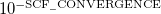. Adjust the value of THRESH at the same time. Note that in Q-Chem 3.0 the DIIS error is measured by the maximum error rather than the RMS error. TYPE:  INTEGER DEFAULT:  5 For single point energy calculations. 7 For geometry optimizations and vibrational analysis. 8 For SSG calculations, see Chapter 5. OPTIONS:Corresponding to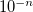RECOMMENDATION:  Tighter criteria for geometry optimization and vibration analysis. Larger values provide more significant figures, at greater computational cost. In some cases besides the total SCF energy, one needs its separate energy components, like kinetic energy, exchange energy, correlation energy, etc. The values of these components are printed at each SCF cycle if one specifies in the input: SCF_PRINT 1 . ## 4.5.3 Direct Inversion in the Iterative Subspace (DIIS) The SCF implementation of the Direct Inversion in the Iterative Subspace (DIIS) method [163, 164] uses the property of an SCF solution that requires the density matrix to commute with the Fock matrix: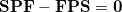(4.75) During the SCF cycles, prior to achieving self-consistency, it is therefore possible to define an error vector e, which is non-zero except at convergence: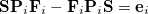(4.76) Here, Pis obtained from diagonalization of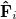, and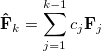(4.77) The DIIS coefficients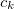, are obtained by a least-squares constrained minimization of the error vectors, viz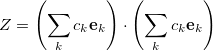(4.78) where the constraint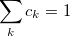(4.79) is imposed to yield a set of linear equations, of dimension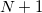: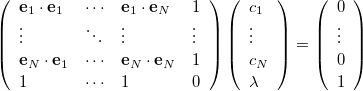(4.80) Convergence criteria requires the largest element of the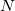th error vector to be below a cutoff threshold, usuallyfor single point energies, often increased to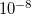for optimizations and frequency calculations. The rate of convergence may be improved by restricting the number of previous Fock matrices (size of the DIIS subspace,$rem variable DIIS_SUBSPACE_SIZE) used for determining the DIIS coefficients: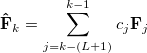(4.81)

where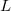is the size of the DIIS subspace. As the Fock matrix nears self-consistency, the linear matrix equations in Eq. (4.80) tend to become severely ill-conditioned and it is often necessary to reset the DIIS subspace (this is automatically carried out by the program).

Finally, on a practical note, we observe that DIIS has a tendency to converge to global minima rather than local minima when employed for SCF calculations. This seems to be because only at convergence is the density matrix in the DIIS iterations idempotent. On the way to convergence, one is not on the “true” energy surface, and this seems to permit DIIS to “tunnel” through barriers in wavefunction space. This is usually a desirable property, and is the motivation for the options that permit initial DIIS iterations before switching to direct minimization to converge to the minimum in difficult cases.

The following $rem variables permit some customization of the DIIS iterations: DIIS_SUBSPACE_SIZE  Controls the size of the DIIS and/or RCA subspace during the SCF. TYPE:  INTEGER DEFAULT:  15 OPTIONS:  User-defined RECOMMENDATION:  None DIIS_PRINT  Controls the output from DIIS SCF optimization. TYPE:  INTEGER DEFAULT:  0 OPTIONS:  0 Minimal print out. 1 Chosen method and DIIS coefficients and solutions. 2 Level 1 plus changes in multipole moments. 3 Level 2 plus Multipole moments. 4 Level 3 plus extrapolated Fock matrices. RECOMMENDATION:  Use default Note: In Q-Chem 3.0 the DIIS error is determined by the maximum error rather than the RMS error. For backward compatibility the RMS error can be forced by using the following$rem

DIIS_ERR_RMS
 Changes the DIIS convergence metric from the maximum to the RMS error.

TYPE:
 LOGICAL

DEFAULT:
 FALSE

OPTIONS:
 TRUE, FALSE

RECOMMENDATION:
 Use default, the maximum error provides a more reliable criterion.

## 4.5.4 Geometric Direct Minimization (GDM)

Troy Van Voorhis, working at Berkeley with Martin Head-Gordon, has developed a novel direct minimization method that is extremely robust, and at the same time is only slightly less efficient than DIIS. This method is called geometric direct minimization (GDM) because it takes steps in an orbital rotation space that correspond properly to the hyper-spherical geometry of that space. In other words, rotations are variables that describe a space which is curved like a many-dimensional sphere. Just like the optimum flight paths for airplanes are not straight lines but great circles, so too are the optimum steps in orbital rotation space. GDM takes this correctly into account, which is the origin of its efficiency and its robustness. For full details, we refer the reader to Ref. VanVoorhis:2002a. GDM is a good alternative to DIIS for SCF jobs that exhibit convergence difficulties with DIIS.

Recently, Barry Dunietz, also working at Berkeley with Martin Head-Gordon, has extended the GDM approach to restricted open-shell SCF calculations. Their results indicate that GDM is much more efficient than the older direct minimization method (DM).

In section 4.5.3, we discussed the fact that DIIS can efficiently head towards the global SCF minimum in the early iterations. This can be true even if DIIS fails to converge in later iterations. For this reason, a hybrid scheme has been implemented which uses the DIIS minimization procedure to achieve convergence to an intermediate cutoff threshold. Thereafter, the geometric direct minimization algorithm is used. This scheme combines the strengths of the two methods quite nicely: the ability of DIIS to recover from initial guesses that may not be close to the global minimum, and the ability of GDM to robustly converge to a local minimum, even when the local surface topology is challenging for DIIS. This is the recommended procedure with which to invoke GDM (i.e., setting SCF_ALGORITHM = DIIS_GDM). This hybrid procedure is also compatible with the SAD guess, while GDM itself is not, because it requires an initial guess set of orbitals. If one wishes to disturb the initial guess as little as possible before switching on GDM, one should additionally specify MAX_DIIS_CYCLES = 1 to obtain only a single Roothaan step (which also serves up a properly orthogonalized set of orbitals).

The MOM-related $rem variables in full are the following:. MOM_PRINT  Switches printing on within the MOM procedure. TYPE:  LOGICAL DEFAULT:  FALSE OPTIONS:  FALSE Printing is turned off TRUE Printing is turned on. RECOMMENDATION:  None MOM_START  Determines when MOM is switched on to stabilize DIIS iterations. TYPE:  INTEGER DEFAULT:  0 (FALSE) OPTIONS:  0 (FALSE) MOM is not usedMOM begins on cycle. RECOMMENDATION:  Set to 1 if preservation of initial orbitals is desired. If MOM is to be used to aid convergence, an SCF without MOM should be run to determine when the SCF starts oscillating. MOM should be set to start just before the oscillations. ## 4.5.7 Relaxed Constraint Algorithm (RCA) The relaxed constraint algorithm (RCA) is an ingenious and simple means of minimizing the SCF energy that is particularly effective in cases where the initial guess is poor. The latter is true, for example, when employing a user-specified basis (when the Core or GWH guess must be employed) or when near-degeneracy effects imply that the initial guess will likely occupy the wrong orbitals relative to the desired converged solution. Briefly, RCA begins with the SCF problem as a constrained minimization of the energy as a function of the density matrix,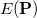[167, 168]. The constraint is that the density matrix be idempotent,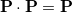, which basically forces the occupation numbers to be either zero or one. The fundamental realization of RCA is that this constraint can be relaxed to allow sub-idempotent density matrices,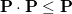. This condition forces the occupation numbers to be between zero and one. Physically, we expect that any state with fractional occupations can lower its energy by moving electrons from higher energy orbitals to lower ones. Thus, if we solve for the minimum ofsubject to the relaxed sub-idempotent constraint, we expect that the ultimate solution will nonetheless be idempotent. In fact, for Hartree-Fock this can be rigorously proven. For density functional theory, it is possible that the minimum will have fractional occupation numbers but these occupations have a physical interpretation in terms of ensemble DFT. The reason the relaxed constraint is easier to deal with is that it is easy to prove that a linear combination of sub-idempotent matrices is also sub-idempotent as long as the linear coefficients are between zero and one. By exploiting this property, convergence can be accelerated in a way that guarantees the energy will go down at every step. The implementation of RCA in Q-Chem closely follows the “Energy DIIS” implementation of the RCA algorithm . Here, the current density matrix is written as a linear combination of the previous density matrices: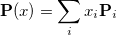(4.82) To a very good approximation (exact for Hartree-Fock) the energy for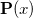can be written as a quadratic function of x: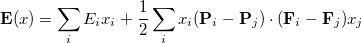(4.83) At each iteration,is chosen to minimize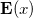subject to the constraint that all of the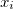are between zero and one. The Fock matrix foris further written as a linear combination of the previous Fock matrices,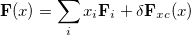(4.84) where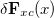denotes a (usually quite small) change in the exchange-correlation part that is computed oncehas been determined. We note that this extrapolation is very similar to that used by DIIS. However, this procedure is guaranteed to reduce the energyat every iteration, unlike DIIS. In practice, the RCA approach is ideally suited to difficult convergence situations because it is immune to the erratic orbital swapping that can occur in DIIS. On the other hand, RCA appears to perform relatively poorly near convergence, requiring a relatively large number of steps to improve the precision of a “good” approximate solution. It is thus advantageous in many cases to run RCA for the initial steps and then switch to DIIS either after some specified number of iterations or after some target convergence threshold has been reached. Finally, note that by its nature RCA considers the energy as a function of the density matrix. As a result, it cannot be applied to restricted open shell calculations which are explicitly orbital-based. Note: RCA interacts poorly with INCDFT, so INCDFT is disabled by default when an RCA or RCA_DIIS calculation is requested. To enable INCDFT with such a calculation, set INCDFT = 2 in the$rem section. RCA may also have poor interactions with INCFOCK; if RCA fails to converge, disabling INCFOCK may improve convergence in some cases.

RCA options are:

RCA_PRINT
 Controls the output from RCA SCF optimizations.

TYPE:
 INTEGER

DEFAULT:
 0

OPTIONS:
 0 No print out 1 RCA summary information 2 Level 1 plus RCA coefficients 3 Level 2 plus RCA iteration details

RECOMMENDATION:
 None

MAX_RCA_CYCLES
 The maximum number of RCA iterations before switching to DIIS when SCF_ALGORITHM is RCA_DIIS.

TYPE:
 INTEGER

DEFAULT:
 50

OPTIONS:
 N N RCA iterations before switching to DIIS

RECOMMENDATION:
 None

THRESH_RCA_SWITCH
 The threshold for switching between RCA and DIIS when SCF_ALGORITHM is RCA_DIIS.

TYPE:
 INTEGER

DEFAULT:
 3

OPTIONS:
 N Algorithm changes from RCA to DIIS when Error is less than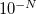.

RECOMMENDATION:
 None

Please see next section for an example using RCA.

## 4.5.8 Small-Gap Systems

SCF calculations for systems with zero or small HOMO-LUMO gap (such as metals) can exhibit very slow convergence or may even fail to converge. This problem arises because the energetic ordering of orbitals and states can switch during the SCF optimization leading to discontinuities in the optimization. Using fractional MO occupation numbers can improve the convergence for small-gap systems. In this approach, the occupation numbers of MOs around the Fermi level are allowed to assume non-integer values. This “occupation smearing” allows one to include multiple electron configurations in the same optimization, which improves the stability of the optimization.

We follow the pseudo-Fractional Occupation Number (pFON) method of Rabuck and Scuseria  that scales the MO occupation used to construct the AO density: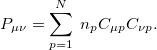(4.85)

For a conventional (integer occupation number) SCF run, the occupation number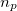is either one (occupied) or zero (virtual). In pFON, the occupation numbers are following a Fermi-Dirac distribution,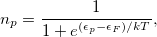(4.86)

where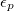is the respective orbital energy and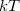the Boltzmann constant and temperature, respectively. The Fermi energy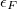is set to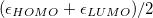in our implementation. To ensure conservation of the total number of electrons, the pFON approach rescales the occupation numbers so that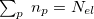.

There are several parameters to control the electronic temperature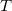throughout a pFON SCF run. The temperature can either be held constant at finite temperature (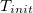=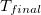), or the system can be cooled from a higher temperature down to the final temperature. So far, no zero-temperature extrapolation has been implemented.

OCCUPATIONS
 Activates pFON calculation.

TYPE:
 INTEGER

DEFAULT:
 0

OPTIONS:
 0 Integer occupation numbers 1 Not yet implemented 2 Pseudo-fractional occupation numbers (pFON)

RECOMMENDATION:
 Use pFON to improve convergence for small-gap systems.

FON_T_START
 Initial electronic temperature (in K) for FON calculation.

TYPE:
 INTEGER

DEFAULT:
 1000

OPTIONS:
 Any desired initial temperature.

RECOMMENDATION:
 Pick the temperature to either reproduce experimental conditions (e.g. room temperature) or as low as possible to approach zero-temperature.

FON_T_END
 Final electronic temperature for FON calculation.

TYPE:
 INTEGER

DEFAULT:
 0

OPTIONS:
 Any desired final temperature.

RECOMMENDATION:
 Pick the temperature to either reproduce experimental conditions (e.g. room temperature) or as low as possible to approach zero-temperature.

FON_NORB
 Number of orbitals above and below the Fermi level that are allowed to have fractional occupancies.

TYPE:
 INTEGER

DEFAULT:
 4

OPTIONS:
 n number of active orbitals

RECOMMENDATION:
 The number of valence orbitals is a reasonable choice.

FON_T_SCALE
 Determines the step size for the cooling.

TYPE:
 INTEGER

DEFAULT:
 90

OPTIONS:
 n temperature is scaled by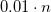in each cycle (cooling method 1) n temperature is decreased by n K in each cycle (cooling method 2)

RECOMMENDATION:
 The cooling rate should be neither too slow nor too fast. Too slow may lead to final energies that are at undesirably high temperatures. Too fast may lead to convergence issues. Reasonable choices for methods 1 and 2 are 98 and 50, respectively. When in doubt, use constant temperature.

FON_E_THRESH
 DIIS error below which occupations will be kept constant.

TYPE:
 INTEGER

DEFAULT:
 4

OPTIONS:
 n freeze occupations below DIIS error ofRECOMMENDATION:
 This should be one or two numbers bigger than the desired SCF convergence threshold.

FON_T_METHOD
 Selects cooling algorithm.

TYPE:
 INTEGER

DEFAULT:
 1

OPTIONS:
 1 temperature is scaled by a factor in each cycle 2 temperature is decreased by a constant number in each cycle

RECOMMENDATION:
 We have made slightly better experience with a constant cooling rate. However, choose constant temperature when in doubt.

## 4.5.9 Examples

Example 4.61  Input for a UHF calculation using geometric direct minimization (GDM) on the phenyl radical, after initial iterations with DIIS. This example fails to converge if DIIS is employed directly.

$molecule 0 2 c1 x1 c1 1.0 c2 c1 rc2 x1 90.0 x2 c2 1.0 c1 90.0 x1 0.0 c3 c1 rc3 x1 90.0 c2 tc3 c4 c1 rc3 x1 90.0 c2 -tc3 c5 c3 rc5 c1 ac5 x1 -90.0 c6 c4 rc5 c1 ac5 x1 90.0 h1 c2 rh1 x2 90.0 c1 180.0 h2 c3 rh2 c1 ah2 x1 90.0 h3 c4 rh2 c1 ah2 x1 -90.0 h4 c5 rh4 c3 ah4 c1 180.0 h5 c6 rh4 c4 ah4 c1 180.0 rc2 = 2.672986 rc3 = 1.354498 tc3 = 62.851505 rc5 = 1.372904 ac5 = 116.454370 rh1 = 1.085735 rh2 = 1.085342 ah2 = 122.157328 rh4 = 1.087216 ah4 = 119.523496$end

$rem BASIS = 6-31G* METHOD = hf INTSBUFFERSIZE = 15000000 SCF_ALGORITHM = diis_gdm SCF_CONVERGENCE = 7 THRESH = 10$end


Example 4.62  An example showing how to converge a ROHF calculation on the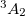state of DMX. Note the use of reading in orbitals from a previous closed-shell calculation and the use of MOM to maintain the orbital occupancies. The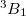is obtained if MOM is not used.

$molecule +1 1 C 0.000000 0.000000 0.990770 H 0.000000 0.000000 2.081970 C -1.233954 0.000000 0.290926 C -2.444677 0.000000 1.001437 H -2.464545 0.000000 2.089088 H -3.400657 0.000000 0.486785 C -1.175344 0.000000 -1.151599 H -2.151707 0.000000 -1.649364 C 0.000000 0.000000 -1.928130 C 1.175344 0.000000 -1.151599 H 2.151707 0.000000 -1.649364 C 1.233954 0.000000 0.290926 C 2.444677 0.000000 1.001437 H 2.464545 0.000000 2.089088 H 3.400657 0.000000 0.486785$end

$rem UNRESTRICTED false METHOD hf BASIS 6-31+G* SCF_GUESS core$end

@@@

$molecule read$end

$rem UNRESTRICTED false METHOD hf BASIS 6-31+G* SCF_GUESS read MOM_START 1$end

$occupied 1:26 28 1:26 28$end

@@@

$molecule -1 3 ... <as above> ...$end

$rem UNRESTRICTED false METHOD hf BASIS 6-31+G* SCF_GUESS read$end


Example 4.63  RCA_DIIS algorithm applied a radical

$molecule 0 2 H 1.004123 -0.180454 0.000000 O -0.246002 0.596152 0.000000 O -1.312366 -0.230256 0.000000$end

$rem UNRESTRICTED true METHOD hf BASIS cc-pVDZ SCF_GUESS gwh SCF_ALGORITHM RCA_DIIS DIIS_SUBSPACE_SIZE 15 THRESH 9$end


Example 4.64  pFON calculation of a metal cluster.

$molecule 0 1 Pt -0.20408 1.19210 0.54029 Pt 2.61132 1.04687 0.66196 Pt 0.83227 0.03296 -1.49084 Pt 0.95832 -1.05360 0.92253 Pt -1.66760 -1.07875 -1.02416$end

$rem jobtyp sp method PBE ecp lanl2dz BASIS lanl2dz Maxscf 2000 symmetry 0 occupations 2 ! pseudo-fractional occupation numbers FON_NORB 10 FON_T_START 300 ! electronic temperature: 300 K FON_T_END 300 FON_E_THRESH 5 ! freeze occupation numbers once DIIS error is 10^-5$end